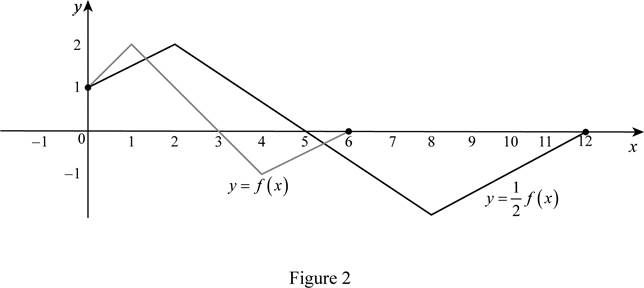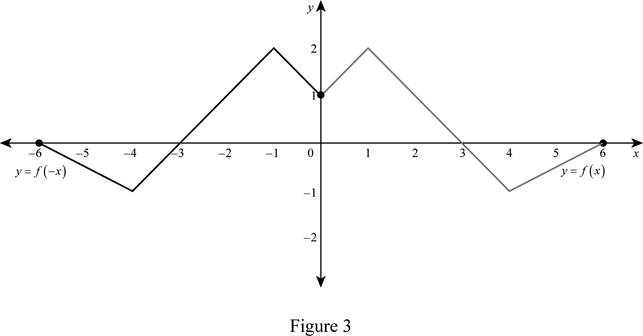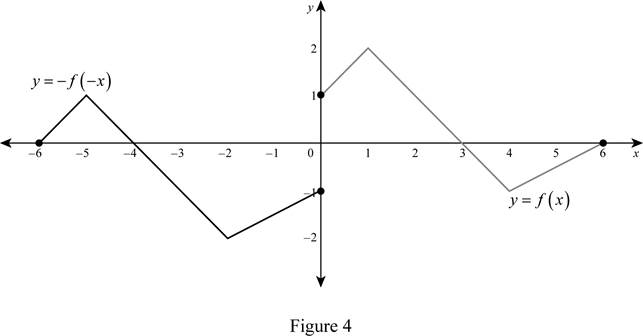# The graph of the function y = f ( 2 x ) by using the given graph.### Single Variable Calculus: Concepts...

4th Edition
James Stewart
Publisher: Cengage Learning
ISBN: 9781337687805### Single Variable Calculus: Concepts...

4th Edition
James Stewart
Publisher: Cengage Learning
ISBN: 9781337687805

#### Solutions

Chapter 1.3, Problem 5E

(a)

To determine

## To sketch: The graph of the function y=f(2x) by using the given graph.

Expert Solution

### Explanation of Solution

The equation y=f(2x) represents that the graph of the function is horizontally shrunk by a factor of 2. Thus, the graph of y=f(2x) is shown below in Figure 1.From Figure 1, it is observed that the actual curve is horizontally shrunk by a factor of 2.

(b)

To determine

Expert Solution

### Explanation of Solution

The equation y=f(12x) represents that the graph of the function is stretched horizontally by a factor of 2. Thus, the graph of y=f(12x) is shown below.From Figure 2, it is observed that the actual curve is stretched horizontally by a factor of 2.

(c)

To determine

Expert Solution

### Explanation of Solution

The equation y=f(x) represents that the graph of the function is reflected about y-axis. Thus, the graph of y=f(x) is shown below in Figure 3.From Figure 3, it is observed that the actual curve is reflected through vertical axis.

(d)

To determine

Expert Solution

### Explanation of Solution

The equation y=f(x) represents that the graph of the function is reflected about the y-axis as well as the x-axis. Thus, the graph of y=f(x) is shown below in Figure 4.From Figure 4, it is observed that the actual curve is reflected about the vertical axis and then the origin.

### Have a homework question?

Subscribe to bartleby learn! Ask subject matter experts 30 homework questions each month. Plus, you’ll have access to millions of step-by-step textbook answers!